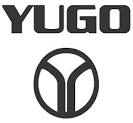# Yugo Workshop Manuals

< PREV PAGE NEXT PAGE >
Page 1Technical Service Bulletin # ATRATB8923

Date: 890801

A/T - Math Formulas Part I

TSB: 89-23 (Aug)

SUBJECT:
TRANSMISSION MATH FORMULAS (Your most valuable tool)

Transmission math formulas are not reserved exclusively for engineers. Understanding some basic mathematical formulas can be one of your most
valuable tools.

The following information contained in this bulletin will discuss various basic formulas dealing with:

1.

Shift Speed

2.

Pressure

3.

Speedometer ratios

Take the time, now, to understand these relatively simple concepts.

You will be saving yourself many problems, and considerable frustration, and also dollars, in the future.

Shift Speed and Pressure

SHIFT SPEED AND PRESSURE

To figure the area of a circle (valve or servo):

Radius (which is 1/2 the diameter) x Radius x 3.14159 = Area

EXAMPLE: A 1" diameter circle has a radius of 0.5"

0.5 x 0.5 x 3.14159 = 0.785

Therefore a 1" diameter circle has an Area of 0.785 sq. inches

Pressure x Area = Force

EXAMPLE: 100 psi line pressure, on a servo with an area of 2 square inches = force

So, 100 psi line pressure x 2 sq in = 200 pounds of force.

Force divided by Area = Pressure

EXAMPLE: 200 lbs divided by 2" = 100 psi

Force divided by Pressure = Area

EXAMPLE: 200 lbs divided by 100 psi = 2 inches

THINGS WE CAN DO WITH THESE FORMULAS:

EXAMPLE: A 700 R4 has 62 psi of line pressure at Idle.
The PR spring weighs 6.5 lbs

The tip (reaction end) of the PR valve has a diameter of 0.365" (0.365 divided by 2 = 0.1825 radius) 0.1825 x 0.1825 x 3.14159 = 0.1046" area

We want 75 psi of line pressure at Idle

First, let's see if those numbers add up, using: Pressure x Area = Force

62 psi x 0.1046 = 6.48, or 6 1/2 lb PR Spring

We want 75 psi:
Pressure x Area = Force (Spring) 75 psi x 0.1046 = 7.85 lb spring

What if we put in an 8 lb Spring? Force divided by Area = Pressure

### Transmission and Drivetrain > Automatic Transmission/Transaxle > Component Information > Technical Service Bulletins > Technical Service Bulletins for Automatic Transmission/Transaxle: > Page 800

< PREV PAGE NEXT PAGE >